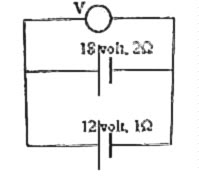## Thursday, August 30, 2007

### Multiple Choice Questions on Direct Current CircuitsThe following MCQ which appeared in All India Pre-Medical/Dental Entrance 2005 Examination (AIPMT) is one requiring the application of Kirchoff’s laws:

Two batteries, one of emf 18 volt and internal resistance 2 Ω and the other of emf 12 volt and internal resistance 1 Ω, are connected as shown. The voltmeter V will record a reading of

(a) 30 volt (b) 18 volt (c) 15 volt (d) 14 volt

The net emf in the closed path containing the two batteries is 18volt – 12 volt = 6 volt

Since this must be equal to the net voltage drop across the internal resistances of the batteries, we have

I(2 + 1) = 6 volt where ‘I’ is the current through the batteries. [Note that the voltmeter will draw negligible current (ideally, zero current) and hence the same current flows through the batteries].

Therefore, I = 2 ampere.

The voltage across the voltmeter is the same as the terminal voltages of the batteries. If you consider the 18 volt battery, its terminal voltage is 18 – (2×2) = 14 volt.

[If you consider the 12 volt battery, its terminal voltage is 12 + (1×2) = 14 volt, which is the same as the above value]. So, the correct option is (d).

Here is another question which you can answer in no time if you had worked out a similar one earlier:

Five identical cells each of emf 1.5 V and internal resistance ‘r’ send the same current through an external resistance of 1 Ω whether the cells are connected in series or in parallel. The internal resistance ‘r’ of each cell is

(a) 0.2Ω (b) 0.5 Ω (c) 1 Ω (d) 1.5 Ω (e) 3 Ω

The condition depicted in the above question occurs when the internal resistance of each cell is equal to the external resistance. The answer therefore is 1 Ω.

The proof is simple: Let there be ‘n’ cells. If the emf of each cell is V and the external resistance is R, the current through R on connecting the cells in series is nV/(nr + R). When the cells are in parallel, the current through R is V/[(r/n) + R].

Since the currents are equal, nV/(nr + R) = V/[(r/n) + R].

Therefore, r + nR = nR + R, from which r = R.

Now, let us consider the following MCQ which appeared in All India Pre-Medical/Dental Entrance 2004 Examination question paper:

Five equal resistances each of resistance R are connected as shown in the figure. A battery of V volts is connected between A and B. The current flowing in AFCEB will be(a) 3V/R (b) V/R (c) V/2R (d) 2V/R

Once you realise that C and D are equipotential points with respect to point A, the resistance R connected directly between C and D can be ignored (since it will not carry any current). The current through the path AFCEB is therefore V/2R.

`Science is a wonderful thing if one does not have to earnone's living at it`
`–Albert Einstein `